Search

About 14 Search Results Matching Types of Worksheet, Worksheet Section, Generator, Generator Section, Kids Sudoku Puzzle3, Subjects matching Fractions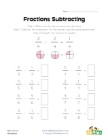Simple Fraction Subtraction Worksheet 1

This fractions worksheet has 8 simple fraction sub...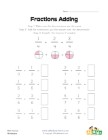Simple Fraction Addition Worksheet

This fractions worksheet has 8 simple fraction add...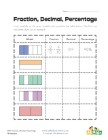Fractions, Decimals and Percentages Worksheet 4

Use the model to figure out the fraction, decimal ...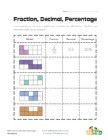Fractions, Decimals and Percentages Worksheet 2

Use the model to figure out the fraction, decimal ...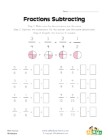Simple Fraction Subtraction Worksheet 2

This worksheet has 8 simple subtracting like fract...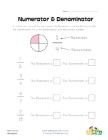Numerator and Denominator Worksheet

Simple worksheet to work on identifying the numera...